## Base Bias with Collector Feedback Circuit:

This Base Bias with Collector Feedback Circuit is like a fixed bias circuit except that the base resistor RB is returned to the collector terminal instead of supply VCC. It derives its name from the fact that voltage for RB is derived from the collector. There exists a negative feedback effect which tends to stabilize IC against changes either as a result of change in temperature or as a result of replacement of the transistor by another one.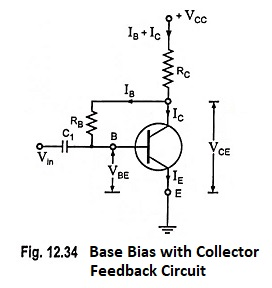Let us see how does this circuit operate. If the collector current IC tends to increase (either due to rise in temperature or due to replacement of transistor), VCE decreases due to larger voltage drop across collector resistor RC. The result is that base current IB is reduced. The reduced base current in turn reduces the original increase in collector current IC. Thus a mechanism exists in the circuit which does not allow collector current IC to increase rapidly.

### Analysis of Base Bias with Collector Feedback Circuit:

The required value of base resistor RB to give the zero signal current IC can be determined as follows :

From the Base Bias with Collector Feedback Circuit diagram shown in Fig. 12.34, applying Kirchhoff’s voltage law to the input circuit, we have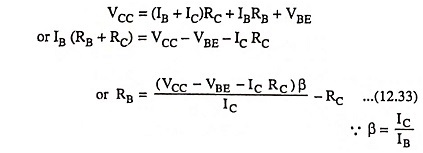From the output section of the circuit we have### Stability Factor S:

From Eq. (12.33)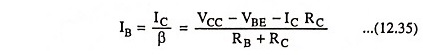Since VBE is almost independent of collector current, so differentiating above equation w.r.t. IC we have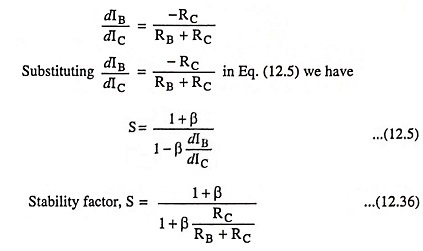Value of stability factor so obtained is less than (1 + β), obtained from fixed bias circuit. So this method provides improved stability as compared to that of a fixed bias circuit.

### Stability Factor SVBE:

From Eq. (12.35)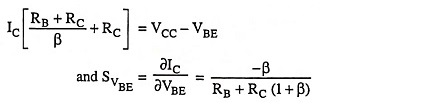Though this Base Bias with Collector Feedback Circuit has a tendency to stabilize the operating point against temperature and β variations but is not much used. The base resistor RB not only provides a dc feedback for the stabilization of the operating point, but it also causes an ac feedback. As a result of this, voltage gain of the amplifier is reduced—an undesirable feature. This is the reason that this circuit is not much used.

Scroll to Top